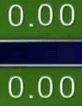# "Counting up" tween number effect?Attempting to recreate the above effect where a number which starts at 0.00 (Yes, decimal included) is animated to whatever value I throw at it;

i.e.
`local EndNumber = 8.18`
or
`local EndNumber = 4.19`

…in such a way that no matter if it is a high or low number, they will arrive at the same time over a period of lets say… x seconds.

I have tried typewriter effect that is very simple and intuitive, but clearly not the effect I am searching for. No idea where to start.1 Like

So, to get the same number of seconds, you’ll need to get an increment amount for every number jump, of course, being different for different numbers.

Then, we need a wait amount for each time it goes up. It has to be very small, but after those increments, the desired number will be reached in the desired number of seconds.

Let’s declare the wait amount to began with:

``````local waitEachTime = 0.1
``````

Then, we’ll create a duration constraint, like 1 second:

``````local dur = 1 --finish in 1 second
``````

Because `1 / 0.1` is 10, we know that the loop has to run 10 times.

``````local cycles = dur / waitEachTime
``````

But, what’s the increment, well that’s where the difference between the two numbers come in:

``````local delta = number2 - number1
--no absolute value, so the order will matter; this will go from number1 to number2
``````

Since the loop runs 10 times, it’ll need to cover this difference within 10 cycles, so each cycle needs to be the difference divided by 10.

``````local inc = delta / cycles
``````

Now, put that into a loop:

``````for i = number1, number2, inc do

--more controlled than intValue.Value = intValue.Value + inc
intValue.Value = number1 + (i * inc)
wait(waitEachTime)

end
``````
Example

Let’s say we want to:

## Go from 0 --> 10 in 2 seconds with 0.5 second wait for each cycle

The delta amount (change in) is `+10` because `10 - 0 = 10`.

The number of cycles is `4` because `2 / 0.5 = 4`.

The increment is simply `+2.5` because `10 / 4 = +2.5`.

Now to test my little formula in the loop is working correctly, let’s manually loop:

cycle (i) value
i = 0 (before loop) 0
i = 1 2.5
i = 2 5
i = 3 7.5
i = 4 10

Surely enough, in 4 cycles we reached 10 and that means that the total wait time is `0.5 * 4`, which is 2, just what we wanted!

Hope that helps!

7 Likes

Thank you for being so in depth, keeping everything to “`get the same number of seconds`” was exactly what I was struggling with.

For anyone curious, I was able to accomplish the desired effect by using `math.floor` and a bit of maths to remove extraneous and lengthy decimals and the result is pretty cool!

2 Likes